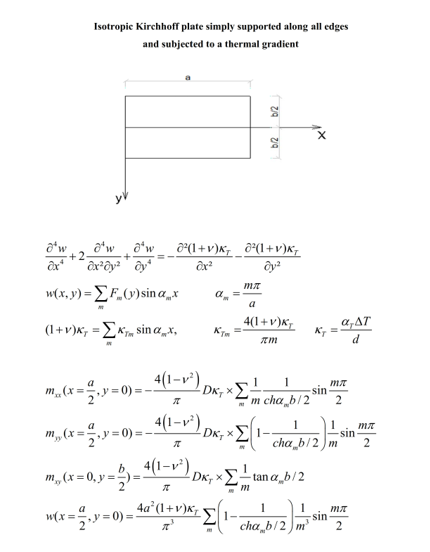Preprint Article Version 1 Preserved in Portico This version is not peer-reviewed

# Bending Analysis of Isotropic Rectangular Kirchhoff Plates Subjected to a Thermal Gradient Using the Fourier Transform Method

Version 1 : Received: 17 June 2021 / Approved: 18 June 2021 / Online: 18 June 2021 (12:52:52 CEST)

How to cite: Fogang, V. Bending Analysis of Isotropic Rectangular Kirchhoff Plates Subjected to a Thermal Gradient Using the Fourier Transform Method . Preprints 2021, 2021060479 (doi: 10.20944/preprints202106.0479.v1). Fogang, V. Bending Analysis of Isotropic Rectangular Kirchhoff Plates Subjected to a Thermal Gradient Using the Fourier Transform Method . Preprints 2021, 2021060479 (doi: 10.20944/preprints202106.0479.v1).

## Abstract

The object of this paper is the bending analysis of isotropic rectangular Kirchhoff plates subjected to a thermal gradient (TG) using the Fourier transform method. The bottom and top surfaces of the plate are assumed to have different changes in temperature, whereas the change in temperature of the mid-surface is zero. According to classical plate theory, the governing equation of the plate contains second derivatives of the TG; these derivatives are zero by constant value of the TG, which leads to the absence of the TG in the governing equation. This paper presented an approach by which Fourier sine transform was utilized to describe the TG, while the double trigonometric series of Navier and the simple trigonometric series of Lévy were utilized to describe the deflection. Thus, the TG appeared in the governing equation, which simplified the analysis. Rectangular plates simply supported along all edges were analyzed, bending moments, twisting moments, and deflections being determined. In addition, rectangular plates simply supported along two opposite edges were analyzed, the other edges having various support conditions (free, simply supported, and fixed).## Subject Areas

Isotropic rectangular plate; Kirchhoff–Love plate theory; thermal gradient; Fourier sine transform; Navier solution; Lévy solution

Views 0